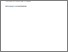Classification of complex systems by their sample-space scaling exponents

Korbel, J., Hanel, R., & Thurner, S. (2018). Classification of complex systems by their sample-space scaling exponents. New Journal of Physics 20 (9) e093007. 10.1088/1367-2630/aadcbe.Preview
Text
Korbel_2018_New_J._Phys._20_093007.pdf - Published Version

Abstract

The nature of statistics, statistical mechanics and consequently the thermodynamics of stochastic systems is largely determined by how the number of states W(N) depends on the size N of the system. Here we propose a scaling expansion of the phasespace volume W(N) of a stochastic system. The corresponding expansion coefficients (exponents) define the universality class the system belongs to. Systems within the same universality class share the same statistics and thermodynamics. For sub-exponentially growing systems such expansions have been shown to exist. By using the scaling expansion this classification can be extended to all stochastic systems, including correlated, constraint and super-exponential systems. The extensive entropy of these systems can be easily expressed in terms of these scaling exponents. Systems with super-exponential phasespace growth contain important systems, such as magnetic coins that combine combinatorial and structural statistics. We discuss other applications in the statistics of networks, aging, and cascading random walks.

Item Type: Article :scaling expansion, extensive entropy, super-exponential systems, complex systems, sample space Advanced Systems Analysis (ASA) Luke Kirwan 15 Oct 2018 07:08 27 Aug 2021 17:30 http://pure.iiasa.ac.at/15529View Item